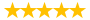# Financial Planning Problems

Question # 00004991 Posted By: vikas Updated on: 12/09/2013 12:42 AM Due on: 12/30/2013
Subject Finance Topic Finance Tutorials:
Question
Question 1
ABC Inc. issued twelve-year, 6 percent semi-annual coupon bonds at par. Today, the bonds are priced at \$1112. What is the firm’s after-tax cost of debt if the tax rate is 30%?
Question 2
If you receive \$1,067 at the end of each year for the first three years and \$5,304 at the end of each year for the next three years. What is the net present value of this cash flow stream? Assume interest rate is 9.2%.
Question 3
The nominal rate is 16% compounded monthly. Compute the effective rate.
Question 4
The risk-free rate is 6.2%, the market risk premium is 8.7%, and the stock’s beta is 1.31. What is the cost of common stock (Ke)?
Question 5
You have a portfolio of two risky stocks which turns out to have no diversification benefit. The reason you have no diversification is the returns:
move perfectly opposite of one another.
are too small.
are completely unrelated to one another.
move perfectly with one another.
are too large to offset.
Question 7
The common stock of ABC Industries is valued at \$47.5 a share. The company increases their dividend by 7.6 percent annually and expects their next dividend to be \$2.29. What is the required rate of return on this stock?
Question 8
You have observed the following returns on ABC's stocks over the last five years:
10.9%, 16%, -6%, 15.8%, 3.6%
What is the arithmetic average returns on the stock over this five-year period.
Question 9
ABC’s last dividend paid was \$0.2, its required return is 13%, its growth rate is 8%, and its growth rate is expected to be constant in the future. What is ABC's expected stock price in 4 years?
Question 10
If the market value of debt is \$137,015, market value of preferred stock is \$54,507, and market value of common equity is 103,045, what is the weight of preferred stock?
Question 11
You would like to create a portfolio that is equally invested in a risk-free asset and two stocks. One stock has a beta of 1.61. What does the beta of the second stock have to be if you want the portfolio to have a beta of 1.19?
Question 12
The ABC Company has a cost of equity of 14.3 percent, a pre-tax cost of debt of 4.8 percent, and a tax rate of 38 percent. What is the firm’s weighted average cost of capital if the weight of debt is 63 percent?
Question 13
Suppose a stock had an initial price of \$16.5 per share, paid a dividend of \$0.9 per share during the year, and had an ending share price of \$19.5. What are the percentage returns if you own 54 shares?
Question 14
You have observed the following returns on ABC's stocks over the last six years:
8.8%, 7.8%, 9.9%, -11.7%, 4.6%, -5.1%
What is the geometric average returns on the stock over this six-year period.
Question 15
A stock just paid a dividend of D0 = \$3.8. The required rate of return is rs = 19.3%, and the constant growth rate is g = 5.8%. What is the current stock price?
Question 16
What is the future value of \$465 at 13% after 9 years?
Question 17
Suppose the real rate is 5.65% and the inflation rate is 5.02%. Solve for the nominal rate.
Question18
How many years will it take for your money to grow from \$537 to \$740 at 6% compounded semi-annually?
An investor puts \$25,000 in a risk-free asset and \$50,000 in the market portfolio. Compute the beta of his portfolio.
0.33
2
0.50
0.67
1
The ABC Co. has \$1,000 face value stock outstanding with a market price of \$934. The stock pays interest annually, matures in 12 years, and has a yield to maturity of 10.6 percent. What is the annual coupon amount?
Suppose that today's stock price is \$43.9. If the required rate on equity is 16.7% and the growth rate is 7.5%, compute the expected dividend (i.e. compute D1)
You want to create a portfolio as risky as the market. Suppose you invest your money in Stocks A, B, C, and the risk-free asset. Compute your investment in Stock C (i.e. solve for weight of Stock C)?
Stock Weights Beta
A 19 1.1
B 15 0.3
C ? 1.6
Rf ? ?
ABC company’s market value of common stock is \$200 million, preferred stock is \$300 million, and debt is \$500 million. Suppose that the cost of equity is 7%, the before-tax cost of debt is 2.7%, cost of preferred stock is 3.9%, and the tax rate is 29%.Compute the WACC.
ABC Company's last dividend was \$2.9. The dividend growth rate is expected to be constant at 9% for 3 years, after which dividends are expected to grow at a rate of 3% forever. The firm's required return (rs) is 17%. What is its current stock price (i.e. solve for Po)?
Tutorials for this Question
1. ## Solution: Financial Planning Problems

Tutorial # 00004779 Posted By: vikas Posted on: 12/09/2013 12:47 AM
Puchased By: 3
Tutorial Preview
par. Today, the bonds are priced at \$1112. What is the ...
Attachments
QA_10.xls (9 KB)
7.docx (12.04 KB)
Recent Feedback
Rated By Feedback Comments Rated On
Sa...hickTutorials helped score A+ in exams 10/12/2014

Great! We have found the solution of this question!